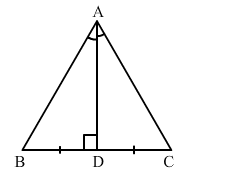# In an equilateral triangle ABC, if AD ⊥ BC, then which of the following is true?`
Question:

In an equilateral triangle ABC, if AD ⊥ BC, then which of the following is true?Solution:

Applying Pythagoras theorem in right-angled triangles ABD and ADC, we get:

$A B^{2}=A D^{2}+B D^{2}$

$\Rightarrow A B^{2}=\left(\frac{1}{2} A B\right)^{2}+A D^{2} \quad\left(\because \triangle \mathrm{ABC}\right.$ is equilateral and $\left.A D=\frac{1}{2} \mathrm{AB}\right)$

$\Rightarrow A B^{2}=\frac{1}{4} A B^{2}+A D^{2}$

$\Rightarrow A B^{2}-\frac{1}{4} A B^{2}=A D^{2}$

$\Rightarrow \frac{3}{4} A B^{2}=A D^{2}$

$\Rightarrow 3 A B^{2}=4 A D^{2}$